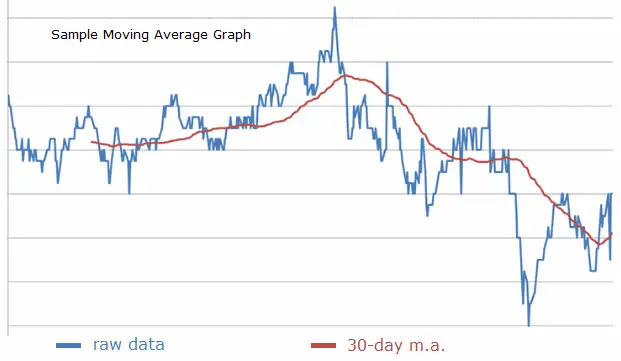# Moving Median Calculator

Given an ordered list of sequential data, you can calculate the n-point moving median by finding the median of each set of n consecutive points in the series. For example, if you have the ordered data set

30, 31, 40, 35, 23, 34, 42, 40, 51,

then the 3-point moving median is

31, 35, 35, 34, 34, 40, 42

Moving medians provide a robust way to smooth sequential data since the median is less sensitive to the effects of outliers than the arithmetic mean. Stock price analysts often look at moving medians long with simple moving averages to see trends more clearly. You can use the calculator below to find the moving median of any data set you input.

 Enter comma-separated data here. DO NOT end list with a comma. Number of Consecutive Points to Average: 2 3 4 5 6 7 8 9 10 11 12 13 14 15 20 21 25 28 30 35 40 50 60 100 Moving Median will be output here.#### Number of Terms in an n-Point Moving Median

Just as with a simple moving average, if the number of terms in the original set is d and the number of data points used in each computation is n, then the number of terms in the moving median sequence will be

d - n + 1.

For example, if you have a sequence of 150 stock prices and take their 7-day moving median, the new resulting sequence will have 150 - 7 + 1 = 144 points.

To compare the moving median with other types of moving averages, see also the simple moving average calculator and the exponential moving average calculator. Both the simple and exponentially weighted moving average are also commonly used in stock price analysis.